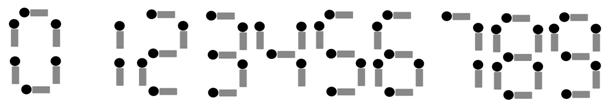Online JudgeProblem SetAuthorsOnline ContestsUser
Web Board
F.A.Qs
Statistical Charts
Problems
Submit Problem
Online Status
Prob.ID:
Register
Authors ranklist
Current Contest
Past Contests
Scheduled Contests
Award Contest
Register
Language:
Matches
 Time Limit: 5000MS Memory Limit: 65536K Total Submissions: 308 Accepted: 34

Description

Zidane is a weird arithmetic expert. Unlike other mathematician, he loves using matches to represent numbers as illustrated in Fig.1.Fig.1

One day Zidane “wrote”an equation on his desk and then went out. The equation was in “A + B = C” format. A, B, and C were numbers made up by matches. “+” and “=” were wrote in ink.

When Zidane came back after a lecture, he found out that somebody must have touched the equation and the equation became wrong! After promised a new toy, Zidane's son confessed that he had broken in the room and moved ONE match (not removed, that match was still in the equation). Given the wrong equation on his desk, can you help Zidane find the original one?

Note: Both the wrong and original equations may have numbers with leading zeros. And it is guaranteed that only ONE possible original equation exists.

Input

The input consists of several test cases. Each test case is a single line representing a wrong equation. Each wrong equation is in the format of “A + B = C” (A, B and C are all numbers), and consists of no more than 100000 characters.

Output

Output the original equation in a single line followed by 17 “*” for each test case.

Sample Input

```0 + 7 = 9
9 + 80 = 8```

Sample Output

```8 + 1 = 9
*****************
8 + 00 = 8
*****************```

Hint

Note: Numbers and operators(“+”, “=”) must be separated by a blank, no mater in the input or in the output.

Source

[Submit]   [Go Back]   [Status]   [Discuss]Home PageGo BackTo top